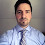## Many developers often stuck while they try to apply range to the Dynamics AX query to filter records based on some conditions. We will try to explore the query ranges in this article and will try to play with some examples to learn how we can apply query ranges using x++ to the Dynamics AX queries. Let’s discussed different cases... Query q; QueryBuildDataSource qbd; QueryBuildRange qbr; q = new Query(); qbd = q.addDataSource(TableNum(CustTable)); qbr = qbd.addRange(FieldNum(CustTable, AccountNum)); qbr.value('4005, 4006'); The above x++ code will generate following SQL statement. "SELECT * FROM CustTable WHERE ((AccountNum = N'4005' OR AccountNum = N'4006'))" qbr.value(strFmt('((AccountNum == "%1") (AccountNum == "%2"))', QueryValue('4005'), QueryValue('4006'))); The above x++ code will generate following SQL statement. "SELECT * FROM CustTable WHERE ((((AccountNum == "4005") || (AccountNum == "4006"))))" Let's say we want to apply "OR" range on a DIFFERENT fields You can use the following x++ code to apply the OR range to the different fields qbr = qbd.addRange(FieldNum(CustTable, DataAreaId)); qbr.value(strFmt('((%1 == "4000") (%2 == "The Bulb"))', fieldStr(CustTable, AccountNum), fieldStr(CustTable, Name))); The above code will generate following sql statement "SELECT * FROM CustTable WHERE ((((AccountNum == "4000") || (Name == "The Axapta"))))" Note: We have used DataAreaId field above to apply the range however, the actual range is on AccountName and AccountNum field. This means when you use range value expressions you can use any field to obtain range object and use it to insert your range in the query. Using DataAreaId field for this purpose is the best practice. -Harry

1.Hello, any idea how to add a range to the 'Having' field value that matches another field?

Essentially this in SQL:

2.Hi,

I need to apply multiple extended query ranges, somewhat similar to your examples above.

When I use DataAreaId to create and apply multiple ranges, I get an OR, which I now understand.